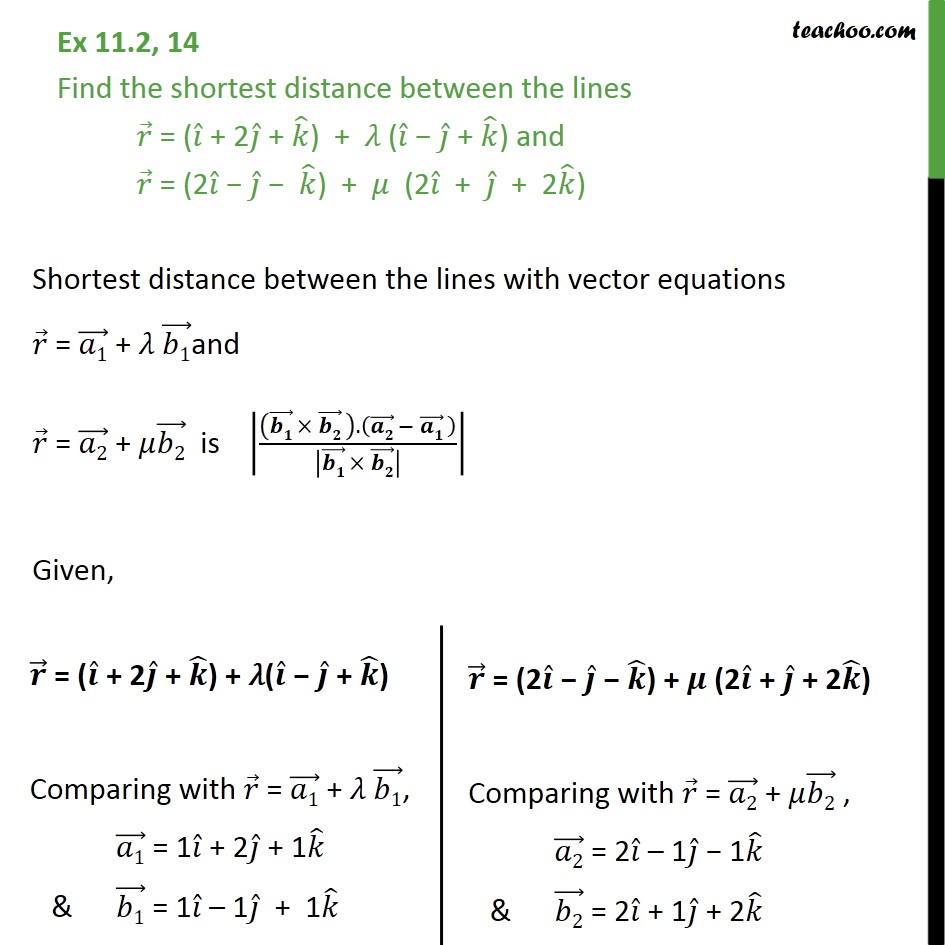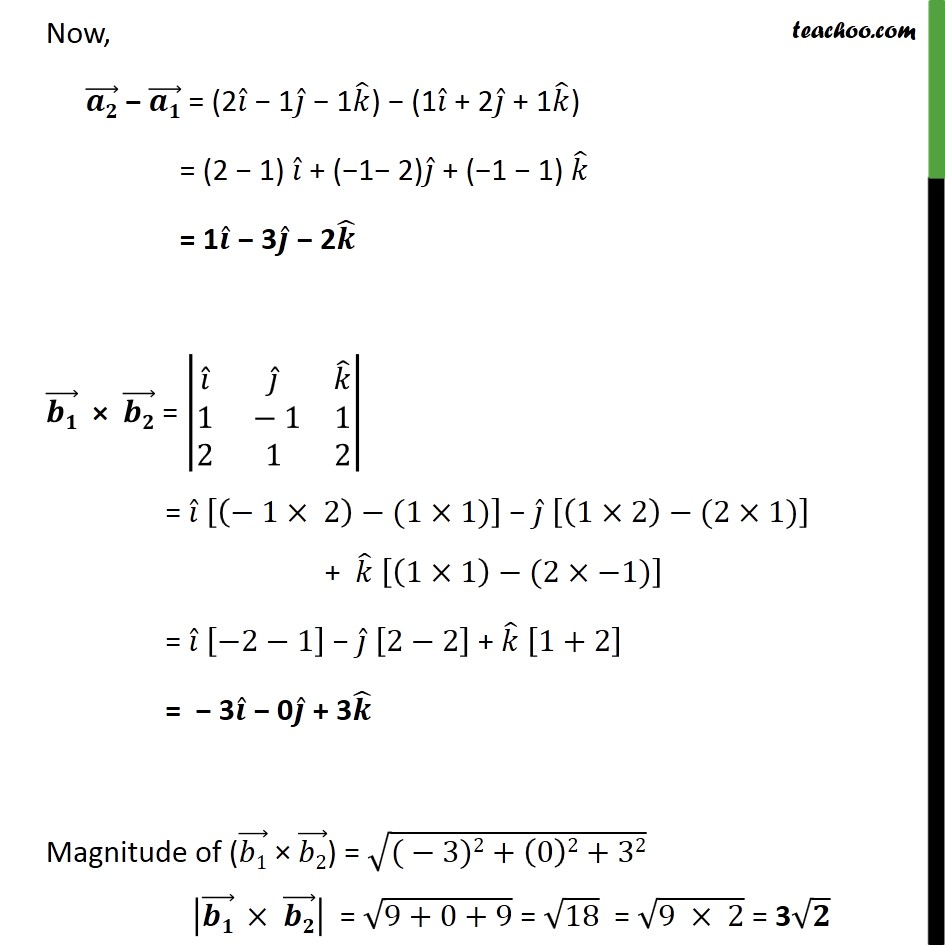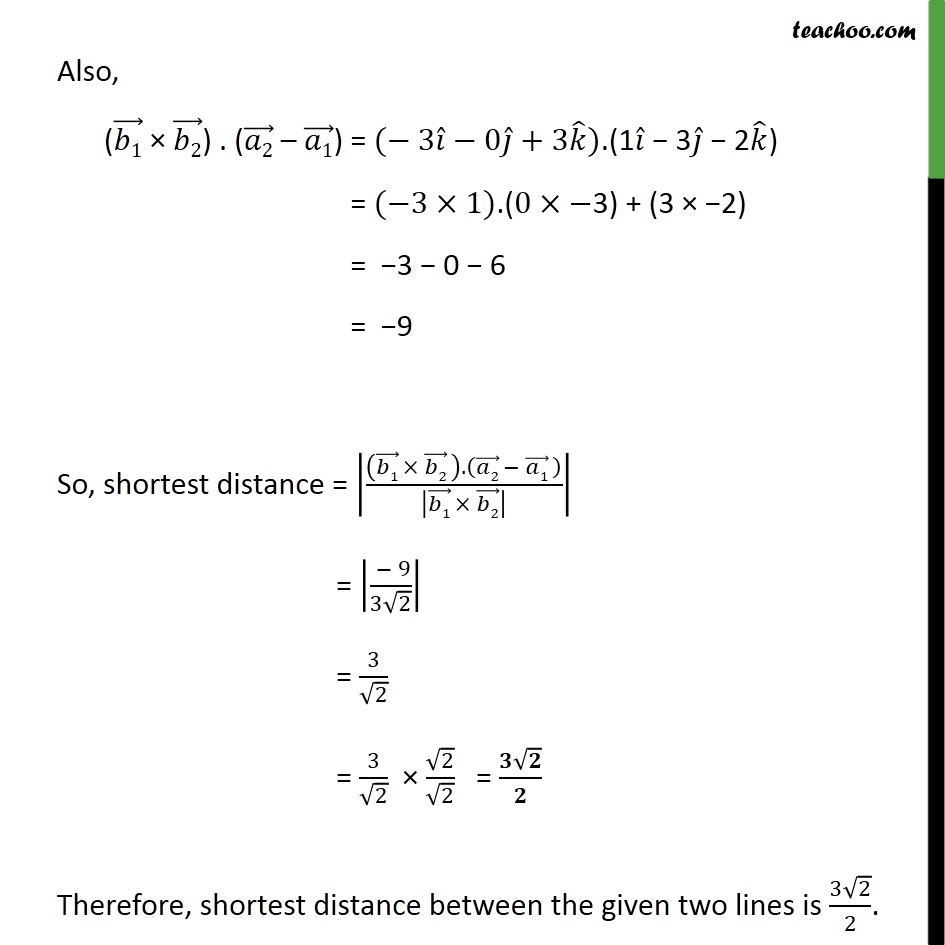1. Chapter 11 Class 12 Three Dimensional Geometry
2. Serial order wise
3. Ex 11.2

Transcript

Ex 11.2, 14 Find the shortest distance between the lines 𝑟﷯ = ( 𝑖﷯ + 2 𝑗﷯ + 𝑘﷯) + 𝜆 ( 𝑖﷯ − 𝑗﷯ + 𝑘﷯) and 𝑟﷯ = (2 𝑖﷯ − 𝑗﷯ − 𝑘﷯) + 𝜇 (2 𝑖﷯ + 𝑗﷯ + 2 𝑘﷯) Shortest distance between the lines with vector equations 𝑟﷯ = 𝑎1﷯ + 𝜆 𝑏1﷯and 𝑟﷯ = 𝑎2﷯ + 𝜇 𝑏2﷯ is 𝒃𝟏﷯ × 𝒃𝟐﷯ ﷯. 𝒂𝟐﷯ − 𝒂𝟏﷯ ﷯﷮ 𝒃𝟏﷯ × 𝒃𝟐﷯﷯﷯﷯ Given, Now, 𝒂𝟐﷯ − 𝒂𝟏﷯ = (2 𝑖﷯ − 1 𝑗﷯ − 1 𝑘﷯) − (1 𝑖﷯ + 2 𝑗﷯ + 1 𝑘﷯) = (2 − 1) 𝑖﷯ + (−1− 2) 𝑗﷯ + (−1 − 1) 𝑘﷯ = 1 𝒊﷯ − 3 𝒋﷯ − 2 𝒌﷯ 𝒃𝟏﷯ × 𝒃𝟐﷯ = 𝑖﷯﷮ 𝑗﷯﷮ 𝑘﷯﷮1﷮ − 1﷮1﷮2﷮1﷮2﷯﷯ = 𝑖﷯ − 1× 2﷯−(1×1)﷯ − 𝑗﷯ 1×2﷯−(2×1)﷯ + 𝑘﷯ 1×1﷯−(2×−1)﷯ = 𝑖﷯ −2−1﷯ − 𝑗﷯ 2−2﷯ + 𝑘﷯ 1+2﷯ = − 3 𝒊﷯ − 0 𝒋﷯ + 3 𝒌﷯ Magnitude of ( 𝑏1﷯ × 𝑏2﷯) = ﷮( − 3)2+ 0﷯2+32﷯ 𝒃𝟏﷯ × 𝒃𝟐﷯﷯ = ﷮9+0+9﷯ = ﷮18﷯ = ﷮9 × 2﷯ = 3 ﷮𝟐﷯ Also, ( 𝑏1﷯ × 𝑏2﷯) . ( 𝑎2﷯ – 𝑎1﷯) = (− 3 𝑖﷯−0 𝑗﷯+3 𝑘﷯).(1 𝑖﷯ − 3 𝑗﷯ − 2 𝑘﷯) = (−3×1).(0×−3) + (3 × −2) = −3 − 0 − 6 = −9 So, shortest distance = 𝑏1﷯ × 𝑏2﷯ ﷯. 𝑎2﷯ − 𝑎1﷯ ﷯﷮ 𝑏1﷯ × 𝑏2﷯﷯﷯﷯ = − 9﷮3 ﷮2﷯﷯﷯ = 3﷮ ﷮2﷯﷯ = 3﷮ ﷮2﷯﷯ × ﷮2﷯﷮ ﷮2﷯﷯ = 𝟑 ﷮𝟐﷯﷮𝟐﷯ Therefore, shortest distance between the given two lines is 3 ﷮2﷯﷮2﷯.

Ex 11.2

About the AuthorDavneet Singh
Davneet Singh is a graduate from Indian Institute of Technology, Kanpur. He has been teaching from the past 9 years. He provides courses for Maths and Science at Teachoo.